##### Multicollinearity# Online Econometrics Textbook - Regression Extensions - Multicollinearity

 When two or more exogenous observation series are highly correlated or have the same predictive power with respect to the endogenous variable they are called multicollinear.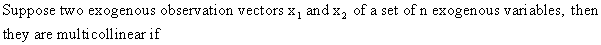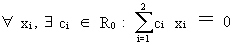(III.IV-1) which means that both vectors are perfectly linearly dependent (see eq. (I.IV-12)). Multicollinearity is a very serious problem, for instance if the researcher is interested in calculating elasticities. If the only aim of the researcher would be to generate forecasts, and if it would be reasonable to assume that the multicollinearity problem would not be different for the forecast period or cross-section, then multicollinearity might be considered not to be a problem at all. This is because multicollinearity will not affect the forecasts of a model but only the weights of the explanatory variables in the model.It is easy to show this mathematically. Assume a multiple regression model with, among others, the two exogenous vectors from (III.IV-1)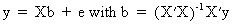(III.IV-2) (see eq. (II.II.1-1) and eq. (II.II.1-3)), then it is obvious to see that X'X must be a non singular symmetric K*K matrix (see property (I.IV-14)). Since we assumed that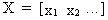(III.IV-3)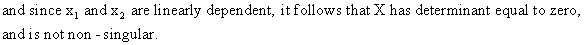For this reason X'X is not non-singular as well, and cannot be inverted (see properties of section I.IV)! Therefore the b vector of the OLS estimation cannot be computed. It should be noted that other estimation methods like GLS, MLE, etc... will also fail. So far we 've discussed the effects of perfect multicollinearity. But what will happen when there is near perfect multicollinearity?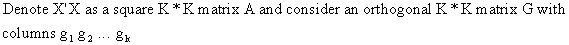(like in eq. (I.IV-51)). The columns are assumed to be the eigenvectors (corresponding to different latent roots) of the square matrix A (see eq. (I.IV-48)). Since GG' = I (see eq. (I.IV-47)) we can write the linear model as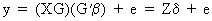(III.IV-4) where the columns of Z are called the principal components, which are orthogonal with respect to each other and have a length equal to their eigenvalue. Since Z is symmetric and pos. semi def., it follows that the eigenvalues are real (eq. (I.IV-37)) and nonnegative (see properties under section I.IV). It can easily be verified that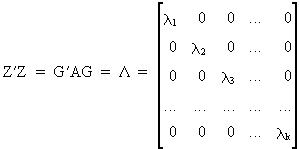(III.IV-5) which follows from (I.IV-54), and agrees with eq. (I.IV-30). Now we are able to write the formula for the parameter vector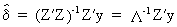(III.IV-6) and more importantly, the parameter covariance matrix and variance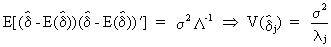(III.IV-7) from which it can be deduced that if the eigenvalue corresponding to a specific exogenous variable becomes small, the respective delta coefficient will have a very large variance. In the extreme case where the eigenvalue were to be zero, the variance would be infinitely large (exact multicollinearity). From (III.IV-4) it follows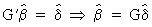(III.IV-8) and from (III.IV-5)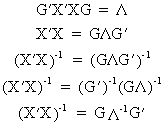(III.IV-9) Now the covariance matrix of the original b parameters can be written as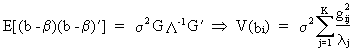(III.IV-10) which not necessarily implies that low values for lambda yield high variances (it also depends on the other parameters). Therefore, in general, all is not lost when lambda is low (but it is possible).No news at the moment...© 2000-2018 All rights reserved. All Photographs (jpg files) are the property of Corel Corporation, Microsoft and their licensors. We acquired a non-transferable license to use these pictures in this website.
The free use of the scientific content in this website is granted for non commercial use only. In any case, the source (url) should always be clearly displayed. Under no circumstances are you allowed to reproduce, copy or redistribute the design, layout, or any content of this website (for commercial use) including any materials contained herein without the express written permission.

Information provided on this web site is provided "AS IS" without warranty of any kind, either express or implied, including, without limitation, warranties of merchantability, fitness for a particular purpose, and noninfringement. We use reasonable efforts to include accurate and timely information and periodically updates the information without notice. However, we make no warranties or representations as to the accuracy or completeness of such information, and it assumes no liability or responsibility for errors or omissions in the content of this web site. Your use of this web site is AT YOUR OWN RISK. Under no circumstances and under no legal theory shall we be liable to you or any other person for any direct, indirect, special, incidental, exemplary, or consequential damages arising from your access to, or use of, this web site.

Contributions and Scientific Research: Prof. Dr. E. Borghers, Prof. Dr. P. Wessa
Please, cite this website when used in publications: Xycoon (or Authors), Statistics - Econometrics - Forecasting (Title), Office for Research Development and Education (Publisher), http://www.xycoon.com/ (URL), (access or printout date).